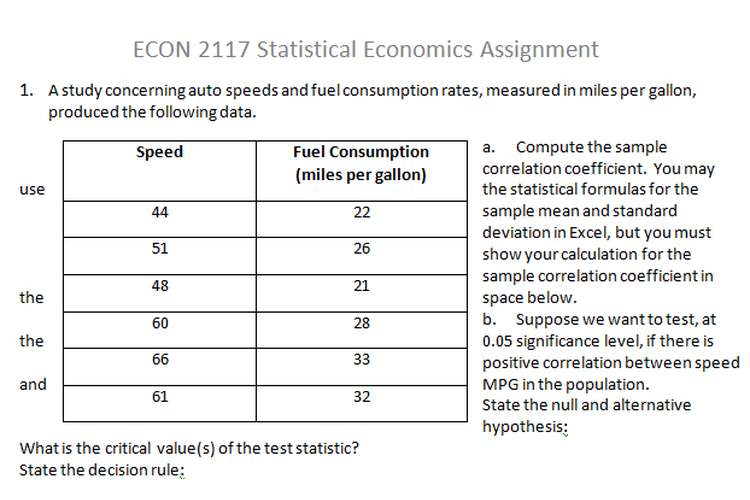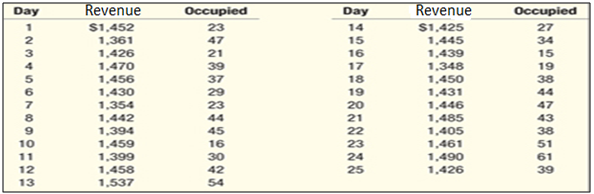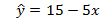ECON 2117 Statistical Economics Editing and Paper Editing ServicesECON 2117 Statistical Economics Editing and Paper Editing Services

This ECON 2117 statistical economics assignment in which we discuss statistical formulas, posotive correlation between speed and MPG in the population.

1. A study concerning auto speeds and fuel consumption rates, measured in miles per gallon, produced the following data.

 Speed Fuel Consumption    (miles per gallon) 44 22 51 26 48 21 60 28 66 33 61 32

a.Compute the sample correlation coefficient.  You may use the statistical formulas for the sample mean and standard deviation in Excel, but you must show your calculation for the sample correlation coefficient in the space below.

b.Suppose we want to test, at the 0.05 significance level, if there is positive correlation between speed and MPG in the population.

State the null and alternative hypothesis
What is the critical value(s) of the test statistic?
State the decision rule:
Compute the value of the test statistic:
Determine and interpret the p-value.

c. Compute the value for theestimated y-intercept and slope, and write out the estimated regression equation.

2. Manuel Rousos, president of Rousos Ford, believes there is a relationship between the number of new cars sold and the number of sales people on duty.  To investigate, he selects a sample of 8 weeks and observes the number of cars sold and the number of sales people on duty for that week as follows:

 Week Sales Staff Cars Sold 1 5 53 2 5 47 3 7 48 4 4 50 5 10 58 6 12 62 7 3 45 8 11 60

a. Use the statistical formula tool in Excel todetermine the value of the sample correlation coefficient.   Based on the value of the correlation coefficient, what can we say about the relationship between sales staff on duty and number of cars sold?
b. Use Excel to generate a scatter chart of the data. Label both axes. Choose the scatter chart option in Excel which shows the estimated regression equation and coefficient of determination. Copy and paste your chart into the space below.
c. What is the estimated population slope? Interpret the value i.e. what does it tell us about the relationship regarding number of sales staff on duty and number of cars sold?
d. What proportion of the variation in number of new cars sold cannot be explained by the number of sales people on duty?
e. Estimate the number of new cars sold when 9 sales people are on duty during a week.
f. Compute a 95% interval for mean car sales for weeks when 9 sales people are on duty.
g. In checking the work schedules for next week, Manuel finds that there are 9sales people scheduled to be on duty.  Develop a 95% interval for the number of cars sold next week.

3. A suburban hotel derives its revenue from its hotel and restaurant operations. The owners are interested in the relationship between the number of rooms occupied on a nightly basis and the revenue per day in the restaurant. Below is a sample of days from last year showing the restaurant revenue and number of rooms occupied.For Excel data file, click on Exercise 13-40Use Data Analysis in Excel (or other software) for the following questions:
Copy and paste your Excel output tables in the space below

1. Write out the estimated regression equation
2. What percent of the variation in restaurant revenue is accounted for by number of rooms occupied?
3. Test to determine if number of rooms occupied is a significant predictor of restaurant revenue at the 5% significance level i.e. test for significance of the slope.

State the null and alternative hypothesis:
State the decision rule:
Compute the value of the test statistic.
Can we conclude that the number of rooms occupied is a significant predictor of restaurant revenue? Explain.
Given the p-value given in the Excel output, would your conclusion be the sameat the 1% level of significance?  Explain.

4. For question number 3, use the statistics generated by Excel data analysis to conduct a hypothesis test to determine if it is reasonable to conclude that there is a linear relationship between restaurant revenue and number of rooms occupied. Use the 5% significance level.

5. The following regression equation was computed from a sample of 20 observations:SSE was found to be 100 and SS total was found to be 400.

1. Complete an ANOVA table based on the above information.
2. Determine the standard error of estimate
3. Determine the sample coefficient of determination
4. Determine the sample correlation coefficient

6. Mike Martin is president of the teachers' union for Lakeview School District. In preparing for upcoming negotiations, he would like to investigate the salary structure of teachers in the district.

He believes there are three factors that influence a teacher's salary: years of experience, a rating of teaching effectiveness given by the principal, and whether or not the teacher has a master's degree. A random sample of 20 teachers resulted in the following data (salary is given in thousands of dollars per year).

a. Copy and paste the Excel summary output in the space below.Write out the estimated regression equation.
b. What is the estimated mean yearly salary for a teacher with 5 years' experience, a rating by the principal of 60, and no master's degree? (Show your work)
c. Conduct a global test to determine if any of the regression coefficients are not equal to zero at the 0.01 level of significance (show all steps of the hypothesis test, along with your concluding statement).
d. Interpret the values generated by Excel for R Square and Standard Error. Is the overall estimated regression model a good one?  Explain with reference to the values for R Square and Standard Error.
e. Should you delete any of the independent variables at the 0.01 significance level? Explain your answer by considering only the p-values generated by Excel.
f. If your decision is to delete one or more independent variables, run the regression analysis again without those variables. Copy and paste your Excel output into the space below. Write out the estimated regression equation when all remaining independent variables are significant.
g.Compare the values for both R Square and Adjusted R Square before and after deleting the insignificant independent variable(s). Why did they increase or decrease? (think about what we would expect to happen to these variables if we had started out with a regression that did not include the insignificant independent variable(s) and then added it to our regression)